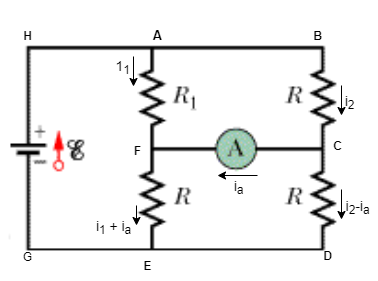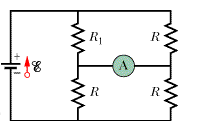# Problem: In Fig. 27-57, R1 = 2.96 R, the ammeter resistance is zero, and the battery is ideal.  What multiple of ε/R gives the current in the ammeter?

###### FREE Expert Solution

Let's label the diagram as follows:From Kirchhoff's loop rule, ΣV = 0

V = iR

If the current is counterclockwise, the potential difference is positive.

If the current is flowing in a clockwise direction, then the potential difference is negative.

Let's apply the Kirchhoff's loop rule to the upper loop ABCFA:

i12.96R - is - i2R = 0

88% (374 ratings)###### Problem Details

In Fig. 27-57, R1 = 2.96 R, the ammeter resistance is zero, and the battery is ideal.  What multiple of ε/R gives the current in the ammeter?